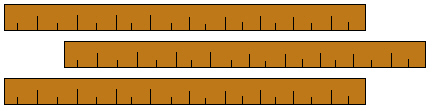Name:    2nd Grade Measurement and Data- Relate addition and subtraction to length, Standard 5, Pre Test 1 Use addition and subtraction within 100 to solve word problems involving lengths that are given in the same units, e.g., by using drawings (such as drawings of rulers) and equations with a symbol for the unknown number to represent the problem.

Multiple Choice
Identify the choice that best completes the statement or answers the question.

1.Emily bought the bar of soap to put in her soap dish.  Which equation below would say how much larger the dish is than the bar of soap?
 a. 4 + 3 = ? c. 4(3) = ? b. 4 x 3 = ? d. 4 - 3 = ?

2.Rhonda used pencil “A” for 3 weeks.  She then got pencil “B,” which was the same size as pencil “A” before she used it.  How many inches of pencil “A” had she used in the 3 weeks?
 a. 3 inches c. 1 inches b. 5 inches d. 2 inches

3.Each of the longer lines on these rulers represents one inch.  How many total inches are on all three rulers?
 a. 36 inches c. 28 inches b. 32 inches d. 30 inches

4.

Alexia was making a dress.  She needed 75 inches of cloth to make the dress.  However, she will have to cut 16 inches off to make it fit perfectly.  How many total inches of cloth will her dress have when it is done?
 a. 60 inches c. 61 inches b. 59 inches d. 55 inches

5.

Sam took a kick-off on the 10 yard line and ran it back 30 yards.  What yard line would the ball be on after Sam’s run?
 a. 20 c. 40 b. 35 d. 50

6.

Petra wanted to race a horse in the 100 meter dash.  Since she was a human, she got a 22 meter head start.  How many meters did Petra have to run in the race?
 a. 78 c. 82 b. 58 d. 73

7.Jack and Jill were racing to fill up their beakers with water by using spoons.  Jack filled beaker “A” and Jill filled beaker “B.”  Each line on the beaker represents 10 milliliters.  How much more water did Jill fill in her beaker than Jack did in his?  Don’t forget to count all the way to the top.
 a. 2 milliliters c. 60 milliliters b. 20 milliliters d. 30 milliliters

8.

Which equation below would solve this problem?
Patrick bought a 50 foot hose to water his garden with.  He wanted it to stretch straight to his garden with no curves.  His garden was 24 feet from his water faucet.  How much did Patrick need to cut off of his hose?
 a. 24 - 50 = ? c. 24 + 50 = ? b. 26 feet d. 50 - 24 = ?

9.

Which equation below would solve this problem?
Allen earned \$16 mowing the lawn.  He then cleaned the garage for another \$56.  How much money did Allen earn?
 a. 16 + 56 = ? c. 16 - 56 = ? b. 56 - 16 = ? d. \$72